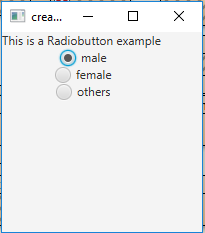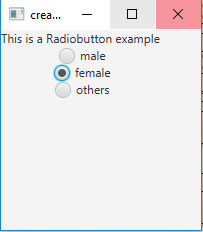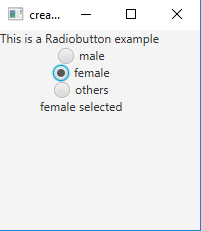Related Articles
• Last Updated : 25 Oct, 2019

RadioButtons are a part of JavaFx package. RadioButtons are mainly used to create a series of items where only one can be selected. When a Radio button is pressed and released an Action event is sent, this Action Event can be handled using an Event Handler.
RadioButton can be added to Toggle Group so that the user cannot select more than one item . By default a radio button is not a part of any toggle group. The selected item of a toggle group can be found using getSelectedToggle() function.

1. RadioButton():Creates a radio button with an empty string for its label.
2. RadioButton(String t):Creates a radio button with the specified text as its label

Commonly used methods:

method explanation
getText() returns the textLabel for radio button
isSelected() returns whether the radiobutton is selected or not
setSelected(boolean b) sets whether the radiobutton is selected or not
setToggleGroup(ToggleGroup tg) sets the toggle group for the radio button
fire() Toggles the state of the radio button if and only if the RadioButton has not already selected or is not part of a ToggleGroup.

Below programs illustrate the RadioButton class:

• Program to create RadioButton and add it to the stage: This program creates a RadioButton indicated by the name r1, r2, r3. The radio button will be created inside a scene, which in turn will be hosted inside a stage (which is the top level JavaFX container). The function setTitle() is used to provide title to the stage. Then a tile-pane is created, on which addChildren() method is called to attach the radio button inside the scene, along with the resolution specified by (200, 200) in the code. Finally, the show() method is called to display the final results.

 `// Java program to create RadioButton and add it to the stage ` `import` `javafx.application.Application; ` `import` `javafx.scene.Scene; ` `import` `javafx.scene.control.*; ` `import` `javafx.scene.layout.*; ` `import` `javafx.event.ActionEvent; ` `import` `javafx.event.EventHandler; ` `import` `javafx.collections.*; ` `import` `javafx.stage.Stage; ` `import` `javafx.scene.text.Text.*; ` `import` `javafx.scene.text.*; ` `public` `class` `radiobutton ``extends` `Application { ` ` `  `    ``// launch the application ` `    ``public` `void` `start(Stage s) ` `    ``{ ` `        ``// set title for the stage ` `        ``s.setTitle(``"creating RadioButton"``); ` ` `  `        ``// create a tile pane ` `        ``TilePane r = ``new` `TilePane(); ` ` `  `        ``// create a label ` `        ``Label l = ``new` `Label(``"This is a Radiobutton example "``); ` ` `  `        ``// create radiobuttons ` `        ``RadioButton r1 = ``new` `RadioButton(``"male"``); ` `        ``RadioButton r2 = ``new` `RadioButton(``"female"``); ` `        ``RadioButton r3 = ``new` `RadioButton(``"others"``); ` ` `  `        ``// add label ` `        ``r.getChildren().add(l); ` `        ``r.getChildren().add(r1); ` `        ``r.getChildren().add(r2); ` `        ``r.getChildren().add(r3); ` ` `  `        ``// create a scene ` `        ``Scene sc = ``new` `Scene(r, ``200``, ``200``); ` ` `  `        ``// set the scene ` `        ``s.setScene(sc); ` ` `  `        ``s.show(); ` `    ``} ` ` `  `    ``public` `static` `void` `main(String args[]) ` `    ``{ ` `        ``// launch the application ` `        ``launch(args); ` `    ``} ` `} `

Output:• Program to create RadioButton and add it to a ToggleGroup: This program creates a RadioButton indicated by the name r1, r2, r3. The radio button will be created inside a scene, which in turn will be hosted inside a stage (which is the top level JavaFX container). The function setTitle() is used to provide title to the stage. A toggle group is created and the radio buttons are added to the toggle group using setToggleGroup() function. Then a tile-pane is created, on which addChildren() method is called to attach the radio button inside the scene, along with the resolution specified by (200, 200) in the code. Finally, the show() method is called to display the final results.

 `// Java Program to create RadioButton and add it to a ToggleGroup ` `import` `javafx.application.Application; ` `import` `javafx.scene.Scene; ` `import` `javafx.scene.control.*; ` `import` `javafx.scene.layout.*; ` `import` `javafx.event.ActionEvent; ` `import` `javafx.event.EventHandler; ` `import` `javafx.collections.*; ` `import` `javafx.stage.Stage; ` `import` `javafx.scene.text.Text.*; ` `import` `javafx.scene.text.*; ` `public` `class` `radiobutton_1 ``extends` `Application { ` `    ``// labels ` `    ``Label l; ` ` `  `    ``// launch the application ` `    ``public` `void` `start(Stage s) ` `    ``{ ` `        ``// set title for the stage ` `        ``s.setTitle(``"creating RadioButton"``); ` ` `  `        ``// create a tile pane ` `        ``TilePane r = ``new` `TilePane(); ` ` `  `        ``// create a label ` `        ``l = ``new` `Label(``"This is a Radiobutton example "``); ` ` `  `        ``// create a toggle group ` `        ``ToggleGroup tg = ``new` `ToggleGroup(); ` ` `  `        ``// create radiobuttons ` `        ``RadioButton r1 = ``new` `RadioButton(``"male"``); ` `        ``RadioButton r2 = ``new` `RadioButton(``"female"``); ` `        ``RadioButton r3 = ``new` `RadioButton(``"others"``); ` ` `  `        ``// add radiobuttons to toggle group ` `        ``r1.setToggleGroup(tg); ` `        ``r2.setToggleGroup(tg); ` `        ``r3.setToggleGroup(tg); ` ` `  `        ``// add label ` `        ``r.getChildren().add(l); ` `        ``r.getChildren().add(r1); ` `        ``r.getChildren().add(r2); ` `        ``r.getChildren().add(r3); ` ` `  `        ``// create a scene ` `        ``Scene sc = ``new` `Scene(r, ``200``, ``200``); ` ` `  `        ``// set the scene ` `        ``s.setScene(sc); ` ` `  `        ``s.show(); ` `    ``} ` ` `  `    ``public` `static` `void` `main(String args[]) ` `    ``{ ` `        ``// launch the application ` `        ``launch(args); ` `    ``} ` `} `

Output:`// Java Program to create RadioButton, add it to a ToggleGroup and add a listener to it ` `import` `javafx.application.Application; ` `import` `javafx.scene.Scene; ` `import` `javafx.scene.control.*; ` `import` `javafx.scene.layout.*; ` `import` `javafx.event.ActionEvent; ` `import` `javafx.event.*; ` `import` `javafx.collections.*; ` `import` `javafx.stage.Stage; ` `import` `javafx.scene.text.Text.*; ` `import` `javafx.scene.text.*; ` `import` `javafx.beans.value.*; ` `public` `class` `radiobutton_2 ``extends` `Application { ` ` `  `    ``// launch the application ` `    ``public` `void` `start(Stage s) ` `    ``{ ` `        ``// set title for the stage ` `        ``s.setTitle(``"creating RadioButton"``); ` ` `  `        ``// create a tile pane ` `        ``TilePane r = ``new` `TilePane(); ` ` `  `        ``// create a label ` `        ``Label l = ``new` `Label(``"This is a Radiobutton example "``); ` `        ``Label l2 = ``new` `Label(``"nothing selected"``); ` ` `  `        ``// create a toggle group ` `        ``ToggleGroup tg = ``new` `ToggleGroup(); ` ` `  `        ``// create radiobuttons ` `        ``RadioButton r1 = ``new` `RadioButton(``"male"``); ` `        ``RadioButton r2 = ``new` `RadioButton(``"female"``); ` `        ``RadioButton r3 = ``new` `RadioButton(``"others"``); ` ` `  `        ``// add radiobuttons to toggle group ` `        ``r1.setToggleGroup(tg); ` `        ``r2.setToggleGroup(tg); ` `        ``r3.setToggleGroup(tg); ` ` `  `        ``// add label ` `        ``r.getChildren().add(l); ` `        ``r.getChildren().add(r1); ` `        ``r.getChildren().add(r2); ` `        ``r.getChildren().add(r3); ` `        ``r.getChildren().add(l2); ` ` `  `        ``// create a scene ` `        ``Scene sc = ``new` `Scene(r, ``200``, ``200``); ` ` `  `        ``// add a change listener ` `        ``tg.selectedToggleProperty().addListener(``new` `ChangeListener()  ` `        ``{ ` `            ``public` `void` `changed(ObservableValue ob,  ` `                                                    ``Toggle o, Toggle n) ` `            ``{ ` ` `  `                ``RadioButton rb = (RadioButton)tg.getSelectedToggle(); ` ` `  `                ``if` `(rb != ``null``) { ` `                    ``String s = rb.getText(); ` ` `  `                    ``// change the label ` `                    ``l2.setText(s + ``" selected"``); ` `                ``} ` `            ``} ` `        ``}); ` ` `  `        ``// set the scene ` `        ``s.setScene(sc); ` ` `  `        ``s.show(); ` `    ``} ` ` `  `    ``public` `static` `void` `main(String args[]) ` `    ``{ ` `        ``// launch the application ` `        ``launch(args); ` `    ``} ` `} `

Output:• Note: The above programs will not run in an online IDE please use an offline compiler